# How to fill NAN values with mean in Pandas?

It is a quite compulsory process to modify the data we have as the computer will show you an error of invalid input as it is quite impossible to process the data having ‘NaN’ with it and it is not quite practically possible to manually change the ‘NaN’ to its mean. Therefore, to resolve this problem we process the data and use various functions by which the ‘NaN’ is removed from our data and is replaced with the particular mean and ready to be processed by the system.

## Python Fill NAN Values With Mean in Pandas

Below are the ways by which we can fill NAN values with mean in Pandas in Python:

• Using Dataframe.fillna() Function
• Using SimpleImputer from sklearn.impute

### Fill NAN Values With Mean in Pandas Using Dataframe.fillna()

With the help of Dataframe.fillna()  from the pandas’ library, we can easily replace the ‘NaN’ in the data frame.

Example 1: Handling Missing Values Using Mean Imputation

In this example, a Pandas DataFrame, ‘gfg,’ is created from a dictionary (‘GFG_dict’) with NaN values in the ‘G2’ column. The code computes the mean of the ‘G2’ column and replaces the NaN values in that column with the calculated mean, resulting in an updated DataFrame.

## Python3

 `import` `numpy as np` `import` `pandas as pd`   `# A dictionary with list as values` `GFG_dict ``=` `{``'G1'``: [``10``, ``20``, ``30``, ``40``],` `            ``'G2'``: [``25``, np.NaN, np.NaN, ``29``],` `            ``'G3'``: [``15``, ``14``, ``17``, ``11``],` `            ``'G4'``: [``21``, ``22``, ``23``, ``25``]}`   `# Create a DataFrame from dictionary` `gfg ``=` `pd.DataFrame(GFG_dict)`   `# Finding the mean of the column having NaN` `mean_value ``=` `gfg[``'G2'``].mean()`   `# Replace NaNs in column S2 with the` `# mean of values in the same column` `gfg[``'G2'``].fillna(value``=``mean_value, inplace``=``True``)` `print``(``'Updated Dataframe:'``)` `print``(gfg)`

Output: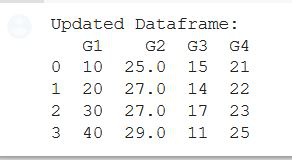Example 2: Filling Mean in NAN Values using Dataframe.fillna()

In this example, a Pandas DataFrame, ‘df,’ is created with missing values in the ‘Sale’ column. The code replaces the NaN values in the ‘Sale’ column with the integer mean of available values, producing an updated DataFrame with filled missing values.

## Python3

 `import` `pandas as pd` `import` `numpy as np`   `df ``=` `pd.DataFrame({` `    ``'ID'``: [``10``, np.nan, ``20``, ``30``, np.nan, ``50``, np.nan,` `           ``150``, ``200``, ``102``, np.nan, ``130``],`   `    ``'Sale'``: [``10``, ``20``, np.nan, ``11``, ``90``, np.nan,` `             ``55``, ``14``, np.nan, ``25``, ``75``, ``35``],`   `    ``'Date'``: [``'2020-10-05'``, ``'2020-09-10'``, np.nan,` `             ``'2020-08-17'``, ``'2020-09-10'``, ``'2020-07-27'``,` `             ``'2020-09-10'``, ``'2020-10-10'``, ``'2020-10-10'``,` `             ``'2020-06-27'``, ``'2020-08-17'``, ``'2020-04-25'``],` `})`   `df[``'Sale'``].fillna(``int``(df[``'Sale'``].mean()), inplace``=``True``)` `print``(df)`

Output: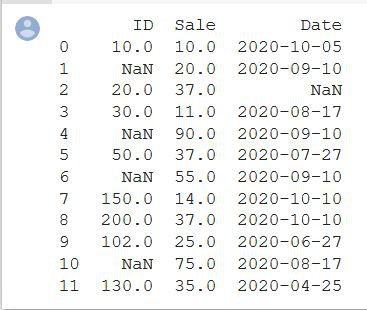### Filling NAN Values With Mean Using SimpleImputer() from sklearn.impute

This function Imputation transformer for completing missing values which provide basic strategies for imputing missing values. These values can be imputed with a provided constant value or using the statistics (mean, median, or most frequent) of each column in which the missing values are located. This class also allows for different missing value encoding.

Syntax: class sklearn.impute.SimpleImputer(*, missing_values=nan, strategy=’mean’, fill_value=None, verbose=0, copy=True, add_indicator=False)

Parameters:

• missing_values: int float, str, np.nan or None, default=np.nan
• strategy string: default=’mean’
• fill_valuestring or numerical value: default=None
• verbose: integer, default=0
• copy: boolean, default=True

Note : Data Used in below examples is here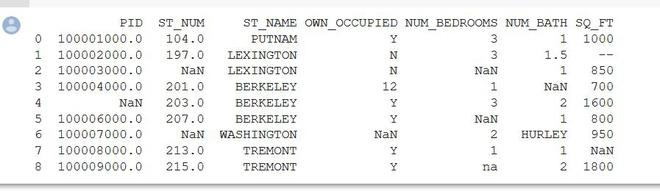Example 1: Computation on PID column

In this example, a property dataset is loaded from a CSV file using Pandas. The code focuses on a specific column (presumably the first column based on `iloc[:, 0]`) denoted as ‘X.’ The `SimpleImputer` from scikit-learn is employed to replace missing values (NaN) in ‘X’ with the mean of the available values, and the updated ‘X’ is printed.

## Python3

 `from` `sklearn.impute ``import` `SimpleImputer` `import` `pandas as pd` `import` `numpy as np`   `Dataset ``=` `pd.read_csv(``"property data.csv"``)` `X ``=` `Dataset.iloc[:, ``0``].values`   `# To calculate mean use imputer class` `imputer ``=` `SimpleImputer(missing_values``=``np.nan, strategy``=``'mean'``)` `imputer ``=` `imputer.fit(X)`   `X ``=` `imputer.transform(X)` `print``(X)`

Output: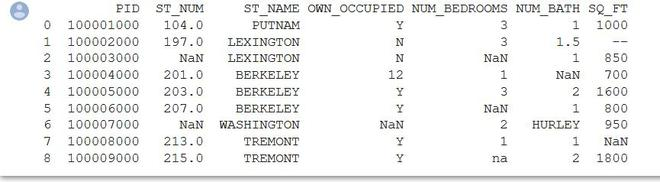Example 2: Computation on ST_NUM Column

In this example, a property dataset is loaded from a CSV file using Pandas. The code focuses on the second column (index 1) denoted as ‘X.’ The `SimpleImputer` from scikit-learn is employed to replace missing values (NaN) in ‘X’ with the mean of the available values.

## Python3

 `from` `sklearn.impute ``import` `SimpleImputer` `import` `pandas as pd` `import` `numpy as np`   `Dataset ``=` `pd.read_csv(``"property data.csv"``)` `X ``=` `Dataset.iloc[:, ``1``].values`   `# To calculate mean use imputer class` `imputer ``=` `SimpleImputer(missing_values``=``np.nan, strategy``=``'mean'``)` `imputer ``=` `imputer.fit(X)` `X ``=` `imputer.transform(X)` `print``(X)`

Output: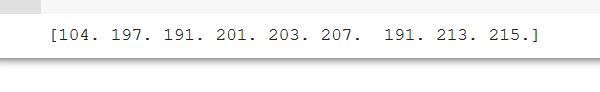Whether you're preparing for your first job interview or aiming to upskill in this ever-evolving tech landscape, GeeksforGeeks Courses are your key to success. We provide top-quality content at affordable prices, all geared towards accelerating your growth in a time-bound manner. Join the millions we've already empowered, and we're here to do the same for you. Don't miss out - check it out now!

Previous
Next